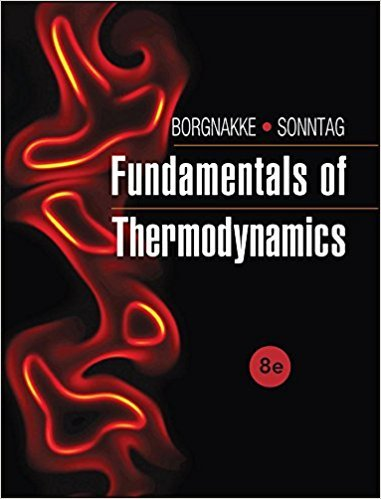×
Get Full Access to Fundamentals Of Thermodynamcs - 8 Edition - Chapter 5 - Problem 7cgp
Get Full Access to Fundamentals Of Thermodynamcs - 8 Edition - Chapter 5 - Problem 7cgp

×

# A combination of two refrigerator cycles is shown in Fig.ISBN: 9781118131992 58

## Solution for problem 7CGP Chapter 5

Fundamentals of Thermodynamcs | 8th Edition

• Textbook Solutions
• 2901 Step-by-step solutions solved by professors and subject experts
• Get 24/7 help from StudySoup virtual teaching assistantsFundamentals of Thermodynamcs | 8th Edition

4 5 1 262 Reviews
27
2
Problem 7CGP

Problem 7CGP

A combination of two refrigerator cycles is shown in Fig. P5.7. Find the overall COP as a function of COP1 and COP2.

FIGURE P5.7Step-by-Step Solution:

Solution 7 CGP

Step 1 of 3

To find the overall Coefficient Of Performance (COP)  for two refrigerator cycles , as a function of Coefficient Of Performance (COP1)  and Coefficient Of Performance (COP2).

Step 2 of 3

Step 3 of 3

##### ISBN: 9781118131992

This textbook survival guide was created for the textbook: Fundamentals of Thermodynamcs , edition: 8. The answer to “A combination of two refrigerator cycles is shown in Fig. P5.7. Find the overall COP as a function of COP1 and COP2.FIGURE P5.7” is broken down into a number of easy to follow steps, and 23 words. Since the solution to 7CGP from 5 chapter was answered, more than 561 students have viewed the full step-by-step answer. Fundamentals of Thermodynamcs was written by and is associated to the ISBN: 9781118131992. This full solution covers the following key subjects: cop, figure, cgp, cycles, fig. This expansive textbook survival guide covers 7 chapters, and 1462 solutions. The full step-by-step solution to problem: 7CGP from chapter: 5 was answered by , our top Engineering and Tech solution expert on 08/03/17, 05:05AM.

Unlock Textbook Solution